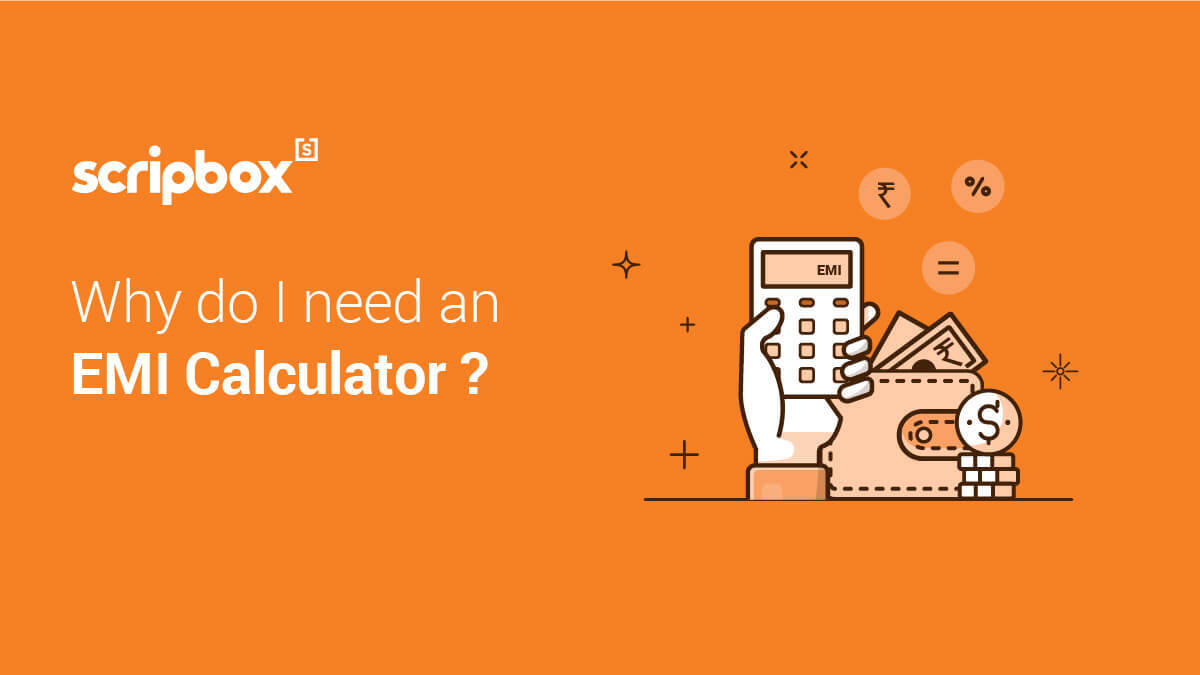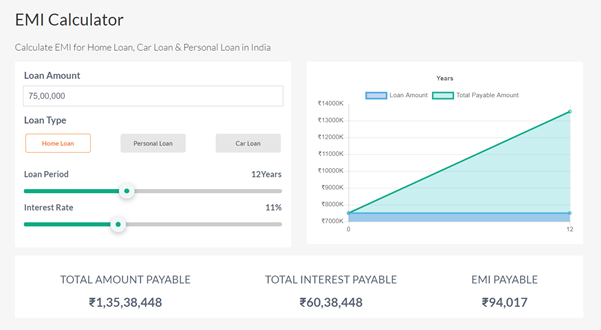# EMI Online Calculator for Home, Car & Personal Loans in India

An equated monthly installment (EMI) is a fixed payment amount made by a borrower to a lender (bank) each month. EMI payment is an integral part of a loan. It is a cumbersome task to determine the EMI amount. By using the EMI calculator, determining the EMI amount is faster and easy.

Year
%

0

0

0

## What is an Equated Monthly Instalment (EMI)?

Equated Monthly Instalment (EMI) is the amount payable to the lender (bank or other financial institution) every month. Through EMIs, the borrower pays the interest and the principal amount each month until total payments of the loan amount is made.A loan is a two-party financial agreement, a lender, and a borrower. The sum of the principal amount and interest is divided by the tenure in which the loan has to be repaid, i.e., number of months. EMI calculator helps in identifying the monthly payouts. EMI payouts are higher in the initial months and gradually decrease with each payment.

## How are Loan EMIs Calculated?

The three most important parameters of calculating a loan EMI are the principal amount, interest on the loan, and the tenure. Each loan EMI varies according to these three parameters. Hence before choosing a particular loan, it is important to have an understanding of these three parameters.

You can calculate your loan EMI of a loan using a mathematical formula, or excel, or online EMI calculator. Of all the ways, the EMI calculator is the easy and fastest way to calculate your loan EMI.

## What is the formula to calculate EMI?

One can calculate EMI using the following formula:Where,

A is EMI Amount

P is Principal Loan Amount

r is the rate of interest for a month

n is loan tenure or duration in number of months

EMI calculators use the above formula to determine the EMI amount. For example, Mr. Ram takes a personal loan of INR 3,00,000 for a tenure of 3 years. The interest rate for his borrowing is 15%. Using the above formula, the calculator determines the EMI payable value as INR 10,400. The total interest payable by Mr. Ram for his borrowing is INR 74,400.

The EMI calculator formula can be applied for different loans. The three variables – loan amount, loan tenure, and the interest rate are the differentiators in the EMI value. The EMI value is directly proportional to the loan amount and interest rate.

In other words, with an increase in the loan amount and interest rate, the EMI value on the loan also increases. However, EMI has an inverse relation with the loan tenure. As the loan tenure increases, the EMI value on the loan decreases and vice versa.

## How a Loan EMI Calculator Works?

The Loan EMI calculator helps in calculating the EMI of a loan within seconds. They convert a tedious calculation into a simple one. The calculator is based on a simple formula:

EMI = P * (r*(1+r)n)/((1+r)n-1)

P is for the Principal.

It is the loan amount given by the lender to the borrower. It can be for a home, a car, or any other reason. The loan amount depends on the cost of the purchase. Higher the amount of the loan higher will be the EMI. The interest is calculated on the principal amount. In the loan EMI calculator, the principal amount has to be entered in the field of 'Loan Amount.'

r is for interest.

It is the rate of interest charged by the lender for lending the principal amount. The interest component of a loan varies from lender to lender and the type of loan. It is always advised to compare interest rates of different lenders of a particular loan before making a choice. In the loan EMI calculator, the interest has to be entered in the field of 'Interest rate.'

n is for loan tenure.

It is the loan repayment time given to repay the loan along with interest. The loan tenure varies with the type of loan, principal amount, and the lender. A car loan can be up to 10 years, but a bank home loan will be longer.

The longer the tenure higher will be the interest paid, and hence higher will be the EMI. In the loan EMI calculator, the tenure has to be entered in the field of the 'Loan Period.

## How to Use Scripbox's Loan EMI Calculator?

Scripbox's Loan EMI calculator is very simple and easy to use. It makes tedious EMI calculations very simple. Scripbox's Loan EMI calculator helps in calculating the principal and interest payable during the tenure and, of course, the loan EMI. The fields in Scripbox's Loan EMI calculator and its usage can be better understood with the help of an example.

Mr. Arun wants to take a bank home loan from a bank for INR 75 lakhs. The interest rate on loan is 11% payable in 12 years. He would like to calculate loan EMI or monthly installment for the loan.

To use the Scripbox's Loan EMI calculator, he will have to enter the data in the following fields:

Loan amount: Mr. Arun's loan amount if INR 75 lakhs

Loan Type: His loan type is a home loan.

Loan Period: The tenure of his loan is 12 years.

Interest rate: The loan interest rate is 11%.

After entering the data in the fields, the calculator returns the following values:

Total amount payable: INR 1,35,38,448

Total interest payable: INR 60,38,448

EMI payable: INR 94,017

Here's a snippet of Scripbox's Loan EMI calculator.Scripbox's Loan EMI calculator works as a car, personal, and a home loan calculator. It helps in calculating the monthly installments to be paid when a loan is taken.

## Types of Loan EMI Calculator

All the calculators work on common parameters. They use the same EMI calculator formula to arrive at the final EMI amount payable. The only difference is the parameters may be subject to a minimum and maximum restriction regarding the type of loan.

Following are the types of Loan EMI Calculators:

### Home loan EMI Calculator

The home loan calculator helps in determining the EMIs to pay for a house loan. While purchasing a home, it requires a high level of planning and research. It is a very important decision both financially and personally due to its long tenure and high loan principal amount.

### Personal Loan EMI Calculator

Personal loans are "all-purpose loans," which means that for any purpose, such as financing a trip or medical expenses. As personal loans are for shorter durations and are unsecured, the interest rate is comparatively higher. Personal Loan Calculator works on the same parameters as other EMI calculators.

### Car loan EMI Calculator

The car loan calculator determines car loan EMIs based upon the loan amount, tenure, and interest rate. For both new and old cars, lenders offer different schemes. This calculator too requires the same details of the loan amount, interest rate, and tenure.

Any down payment paid can be excluded when determining the EMI value using the calculator. If the loan isn't cleared in the stipulated time period, the lender has the right to take the vehicle and auction it off to recover the balance owed.

### Education loan EMI Calculator

Affording good education is becoming difficult as the costs are sky-rocketing. Education loan funds, both domestic and international education. The repayment for an educational loan does not begin immediately but after a moratorium period, which is usually six months after completion of the course or after the student gets a job, whichever is earlier.

### Loan against property EMI calculator

Loan against property is a secured loan that can be used against any property one owns (residential, commercial, or land) that must be mortgaged to the lender. If there are any issues with EMI repayment, the lender has the right to seize the property that has been pledged as collateral.

Business loans are availed to meet various business needs, be it for working capital or any other. Similar to different types of loans, business loans too are repaid through monthly installments. The calculator determines the value of the EMI based on the loan amount, loan tenure, and the interest rate.

## Factors Affecting Loan EMI

Various factors affect the EMI of a loan. Short tenure loans are not affected by these factors, but the long tenure ones like the home loan are surely affected . This is mainly because house loans are linked to MCLR (Marginal Cost of Lending Rate), which keeps changing.

### Change in the interest rate during the loan tenure

Loans are offered either at fixed, floating, or hybrid interest rate regime. In the fixed rate, the interest rate charged is the same throughout the tenure of the loan, and the EMI remains constant too. In floating rates, the interest charged changes throughout the tenure of the loan according to market dynamics so does the EMI amount.

The change can be positive, negative, or there can be no change at all. The hybrid interest rate regime is a mixture of fixed and floating rates. When the loan starts, it is usually fixed interest rate, and later as the tenure passes, the interest rate regime changes to floating. As and when RBI tweaks the standard interest rates, it has an impact on the loan EMI interest rates.

### Prepayment of the Loan Amount

Prepayment of a loan can surely affect the EMI total payments. Most of the banks usually allow prepayment of a loan in full or in part but with some prepayment charges, which are generally around 1-3%.

Any payment made, which is greater than the monthly installment, is considered a prepayment. By prepaying a loan, the principal amount will reduce, and the EMI reduces too. But, prepayment can be done only on certain terms and conditions provided by the lender.

### Change in Loan Tenure

Banks provide their customers to switch the old loan tenure to a new loan tenure similar to home loan balance transfer. The EMI will increase or decrease accordingly.  Change in the tenure has a high impact on the EMI amount. The longer the tenure, the lower is the EMI and shorter the tenure, the higher is the EMI. But the total interest paid is lower in shorter tenure loans than longer tenure loans. Therefore it is very important to consider all the factors, and after using the EMI calculator, one has to decide on changing their loan tenure and balance transfer

### Loans with Flexible Repayment Options

Some banks offer the feature of varying EMIs. These are step-up loans and step-down loans. Step-up loans are loans where the EMI is less during the initial years of the loan and increases gradually with the tenure.

These are suitable for individuals who are in the initial stages of their career cycle. Step-down loans are those where one pays high EMI during the initial years of the loan, and it gradually decreases in the later stages. These are suitable for individuals who are closer to their retirement.

## How to apply online for a loan through internet banking?

Yes, you can apply online for a loan. You must follow the below steps